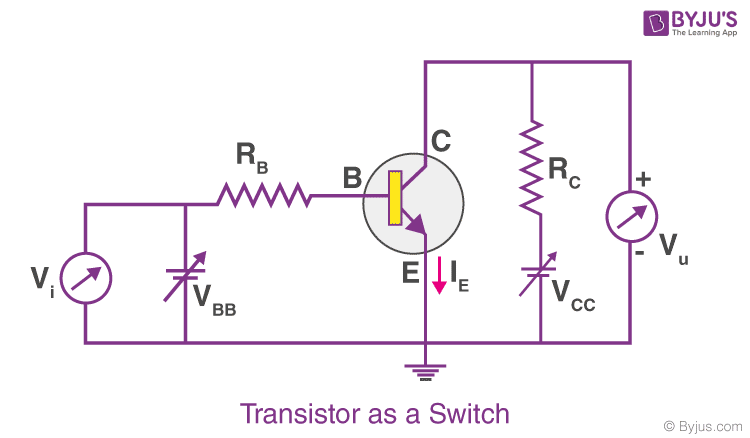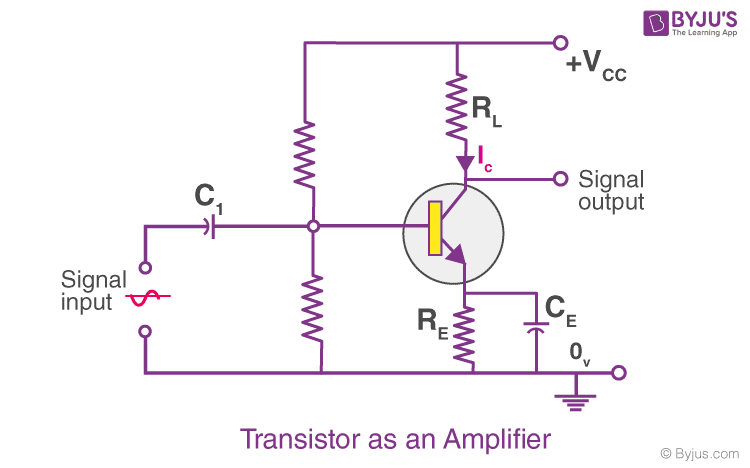# Transistor as a device - Switch and Amplifier

We have read about the configurations in which the transistor can be connected; namely CB, CC, and CE, the biasing of the E-B and B-C junction, and the regions of operations; namely cutoff, the active region, and the saturation region.  We also know that the transistor when used in the cutoff or saturation state, acts as a switch and then operated in the active region is used as an amplifier. In this piece of article, we will learn how a transistor is used as a switch and as an amplifier, in detail.

## Transistor as a SwitchWhen a transistor is used as a switch, there are two operating regions, and they are saturation region where the transistor is fully ON and the cut-off region where the transistor is fully OFF.

Read More: Characteristics of a Transistor

### Cut-off Region

When the transistor is in the cut-off region, this is because there is no flow of current through the transistor. This also means that the base current IB is zero and the collector current IC is also zero. When these currents are zero, the collector voltage VCE would be maximum which results in a large depletion layer.

### Saturation Region

In this region maximum base current, IB is applied to the transistor and the maximum current is obtained at the collector, IC. Since the current is maximum, the voltage at the collector would be minimum. Therefore, in this condition, the transistor is said to be full ON.

Let us consider a base-biased transistor in the CE configuration, as shown in the figure. As we apply Kirchhoff’s voltage law to the input side and the output side of the circuit, we can write,

VBB = IBRB + VBE
VCE = VCC – ICRC

Here VBB is the dc input voltage and VCE is the DC output voltage. Denoting the input voltage as Vi and the output voltage as Vo, we can write it as, Vi = IBRB + VBE, and V0 = VCC – ICRC.
Now, we will see how V0 changes as we increase the Vi. In the case of a Si-transistor, we note that as long as the input voltage is less than 0.6 V, the transistor remains in the cut-off state and the current I is zero, and hence we can write, V0 = VCC.

And when the input voltage Vi is greater than 0.6 V the transistor remains in its active state and the current Ic is obtained in the output path. Also, the output voltage V0 decreases as we increase the ICRC.

Here, as long as the Vi is low and unable to forward-bias the transistor, the value of V0 is high. As soon as the value of Vi becomes high enough such that the transistor attains a saturation state, the value of V0 decreases to a very low value, which is nearly equal to zero. When this transistor is in a state where it is non-conducting, it acts as a switch in its off state and when it is in its saturation state, it acts as a switch in its on the state.

### Applications of transistor as a switch

Following is the list of applications of transistor as a switch:

• The functioning of LED is the most common example of a transistor as a switch.
• The functioning of the DC motors is controlled by the application of different values to the speed of the motor which is further used for turning ON and OFF of the motor.
• Light-dependent resistor uses transistors as a switch.

## Transistor as an AmplifierAs we saw earlier, a transistor in its active state acts as an amplifier, which lies in the active region of the curve between V0 and Vi. In this curve, the slope of the linear part represents the rate at which the signal output changes with respect to the signal input. We can say that the rate is negative as the output is not just ICRC but VCC – ICRC, which is why as we increase the input voltage of the CE amplifier, the output voltage decreases. Here, the output is out of phase with the input signal. Now, if we write the small changes in the output voltage and the input voltages as ΔVo and ΔVi correspondingly, then the ratio of the output signal to the input signal gives the gain in the signal. We can write,

V0 = VCC – ICRC

Therefore, we can write,
ΔV0 = 0 – RCΔIC

As we also have,
Vi = IBRB + VBE

Therefore, we can also write,

ΔVi = RBΔ IB + ΔVBE

But ΔVBE is negligibly small in comparison to ΔIBRB in this circuit. So, the voltage gain of this CE amplifier is given by

$$\begin{array}{l}A_{v}=-\frac{R_{C}\Delta I_{C}}{R_{B}\Delta I_{B}}=-\beta _{ac}\frac{R_{C}}{R_{B}}\end{array}$$

Here, βac is can be given as,

$$\begin{array}{l}\beta _{ac}=\frac{\Delta I_{C}}{\Delta I_{B}}\end{array}$$

We can conclude that the linear portion of the active region of the above-mentioned curve can be used as an amplifier.

## Performance of Amplifier

We know that the most preferred mode of connection is the common emitter mode. When a transistor is used as an amplifier, the performance of the amplifier becomes important. Following are the terms that are used for describing the performance of the amplifier:

### Input Resistance

The input resistance would be low since the input circuit is forward biased. This is the resistance offered by the emitter-base junction to the flow of the signal. Input resistance is defined as the ratio of small change in the emitter-base voltage to the change in the base current when the emitter-collector voltage is kept constant.

### Output Resistance

The output resistance of the transistor as an amplifier would be very high. The output resistance is defined as the ratio of change in emitter-collector voltage to the change in the collector current when the base current is constant.

### Current Gain

Current gain is defined as the ratio of change in the collector current to the change in the base current. The value of current gain may vary from 20 to 500.

### Voltage Gain

Voltage gain is defined as the ratio of change in output voltage to the change in the input voltage.

### Power Gain

The ratio of output signal power to the input signal power is known as power gain.

## Application of transistor as an amplifier

The main application of transistor as an amplifier is in the field of electronics and communication. Following is the list of these applications:

• Transistor as an amplifier is used in optical fiber communication.
• Since the intensity of the output signal is high, it finds application in long-distance communication.
• The amplification of radio signals is possible because of such amplifiers.
• Amplifiers are used in wireless communication.
• The audio amplification is possible because of the use of amplifiers.

Stay tuned with BYJU’S to learn more about how transistors can be used as a switch and an amplifier, actions of a transistor, types of switches, and other related topics.

Test Your Knowledge On Transistor As Switch Amplifier!